Documentation

# harmonicRatio

Harmonic ratio

## Syntax

``hr = harmonicRatio(audioIn,fs)``
``hr = harmonicRatio(audioIn,fs,Name,Value)``

## Description

example

````hr = harmonicRatio(audioIn,fs)` returns the harmonic ratio of the signal, `audioIn`, over time. Columns of the input are treated as individual channels.```

example

````hr = harmonicRatio(audioIn,fs,Name,Value)` specifies options using one or more `Name,Value` pair arguments.Example: ```hr = harmonicRatio(audioIn,fs,'Window',rectwin(round(fs*0.1)),'OverlapLength',round(fs*0.05))``` returns the harmonic ratio for the audio input signal sampled at `fs` Hz. The harmonic ratio is calculated for 100 ms rectangular windows with 50 ms overlap.```

## Examples

collapse all

Read in an audio file, calculate the harmonic ratio using default parameters, and then plot the results.

```[audioIn,fs] = audioread('RandomOscThree-24-96-stereo-13secs.aif'); audioInMono = mean(audioIn,2); hr = harmonicRatio(audioInMono,fs); t = (0:length(audioInMono)-1)/fs; subplot(2,1,1) plot(t,audioInMono) ylabel('Amplitude') t = linspace(0,size(audioInMono,1)/fs,size(hr,1)); subplot(2,1,2) plot(t,hr) xlabel('Time (s)') ylabel('Harmonic Ratio')```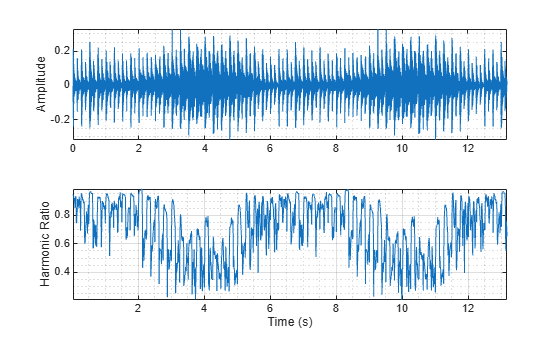`[audioIn,fs] = audioread('Counting-16-44p1-mono-15secs.wav');`

Calculate the harmonic ratio of the audio file using 50 ms Hann windows with 25 ms overlap. Plot the results.

```hr = harmonicRatio(audioIn,fs, ... 'Window',hann(round(fs.*0.05),'periodic'), ... 'OverlapLength',round(fs.*0.025)); t = linspace(0,size(audioIn,1)/fs,size(hr,1)); plot(t,hr) xlabel('Time (s)') ylabel('Harmonic Ratio')```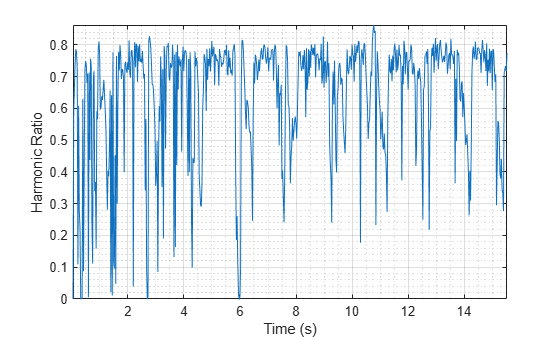The harmonic ratio indicates the ratio of energy in the harmonic portion of audio to the total energy of the audio. Because the audio signal in this example has regions of near silence, where the total energy is very low, the harmonic ratio does a poor job discriminating between regions of speech and regions of silence. Add white noise to the audio signal and then calculate and plot the harmonic ratio.

```audioIn = audioIn + 0.1*randn(size(audioIn)); hr = harmonicRatio(audioIn,fs, ... 'Window',hann(round(fs.*0.05),'periodic'), ... 'OverlapLength',round(fs.*0.025)); t = linspace(0,size(audioIn,1)/fs,size(hr,1)); plot(t,hr) xlabel('Time (s)') ylabel('Harmonic Ratio')```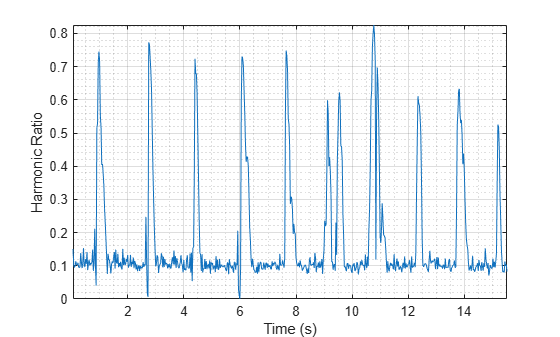Create a `dsp.AudioFileReader` object to read in stereo audio data frame-by-frame. Create a `dsp.SignalSink` object to log the harmonic ratio calculation.

```fileReader = dsp.AudioFileReader('RandomOscThree-24-96-stereo-13secs.aif'); logger = dsp.SignalSink;```

In an audio stream loop:

1. Read in a frame of audio data.

2. Calculate the harmonic ratio for each channel of the frame of audio.

3. Log the harmonic ratio for later plotting.

To calculate the harmonic ratio for only a given input frame, specify a window with the same number of samples as the input, and set the overlap length to zero. Plot the logged data.

```win = hamming(fileReader.SamplesPerFrame,'periodic'); while ~isDone(fileReader) audioIn = fileReader(); hr = harmonicRatio(audioIn,fileReader.SampleRate, ... 'Window',win, ... 'OverlapLength',0); logger(hr) end plot(logger.Buffer) ylabel('Harmonic Ratio') legend('Left Channel','Right Channel')```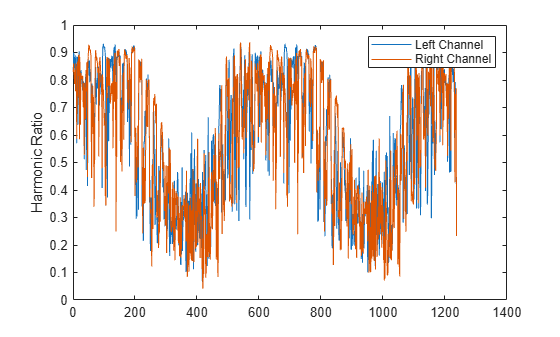If the input to your audio stream loop has a variable samples-per-frame, an inconsistent samples-per-frame with the analysis window size of `harmonicRatio`, or if you want to calculate the harmonic ratio of overlapped data, use `dsp.AsyncBuffer`.

Create a `dsp.AsyncBuffer` object, reset the logger, and release the file reader.

```buff = dsp.AsyncBuffer; reset(logger) release(fileReader)```

Calculate the harmonic ratio using 50 ms frames with a 25 ms overlap.

```fs = fileReader.SampleRate; samplesPerFrame = round(fs*0.05); samplesOverlap = round(fs*0.025); samplesPerHop = samplesPerFrame - samplesOverlap; win = hamming(samplesPerFrame); while ~isDone(fileReader) audioIn = fileReader(); write(buff,audioIn); while buff.NumUnreadSamples >= samplesPerHop audioBuffered = read(buff,samplesPerFrame,samplesOverlap); hr = harmonicRatio(audioBuffered,fs, ... 'Window',win, ... 'OverlapLength',0); logger(hr) end end release(fileReader)```

Plot the logged data.

```plot(logger.Buffer) ylabel('Harmonic Ratio') legend('Left Channel','Right Channel')```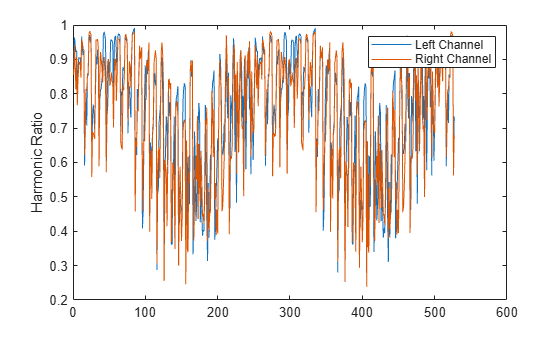The harmonic ratio measures the amount of energy in the tonal part of the signal compared to the amount of energy in the total signal.

Harmonic Ratio of Pure Tone

Create a pure tone and then calculate the harmonic ratio using default parameters. By default, the harmonic ratio is calculated for 30 ms Hamming windows with 10 ms hops. Plot the results. The harmonic ratio is near 1, which is the theoretical maximum.

```fs = 48e3; osc = audioOscillator('Frequency',500, ... 'SamplesPerFrame',192e3,'SampleRate',fs); sinewave = osc(); hr = harmonicRatio(sinewave,fs); t = linspace(0,size(sinewave,1)/fs,size(hr,1)); plot(t,hr) xlabel('Time (s)') ylabel('Harmonic Ratio') title('Sinusoid - Default Parameters')```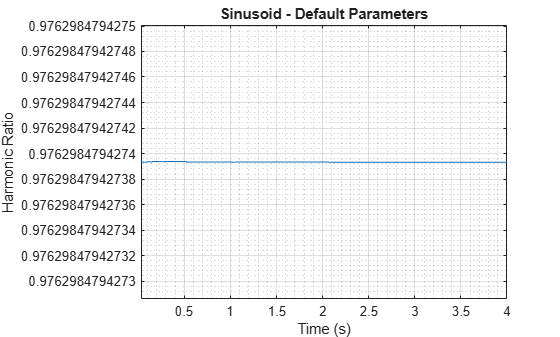The short-time analysis required for windowing lowers the harmonic ratio from the theoretical value of 1. To diminish the effect of windowing, you can increase the window size. Use a 100 ms Hamming window and a 10 ms hop, and observe that the harmonic ratio is closer to one than when using the default window length.

```win = hamming(round(fs*0.1),'periodic'); overlap = round(fs*0.099); hr = harmonicRatio(sinewave,fs,'Window',win,'OverlapLength',overlap); t = linspace(0,size(sinewave,1)/fs,size(hr,1)); plot(t,hr) xlabel('Time (s)') ylabel('Harmonic Ratio') title('Sinusoid - 100 ms Window')```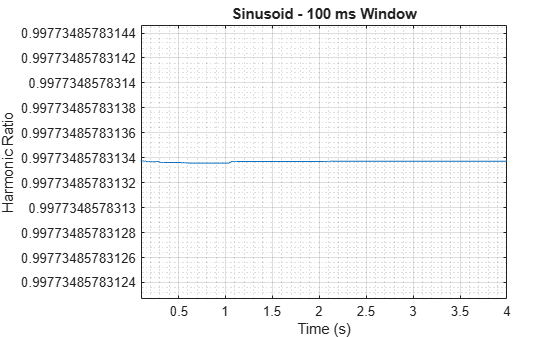Harmonic Ratio of White Noise

Create 5 seconds of white noise and then calculate the harmonic ratio using default parameters. By default, the harmonic ratio is calculated for 30 ms Hamming windows with 10 ms hops. Plot the results. The harmonic ratio is 0.

```fs = 48e3; noise = rand(fs*5,1); hr = harmonicRatio(noise,fs); t = linspace(0,size(noise,1)/fs,size(hr,1)); plot(t,hr) xlabel('Time (s)') ylabel('Harmonic Ratio') title('Noise - Default Parameters')```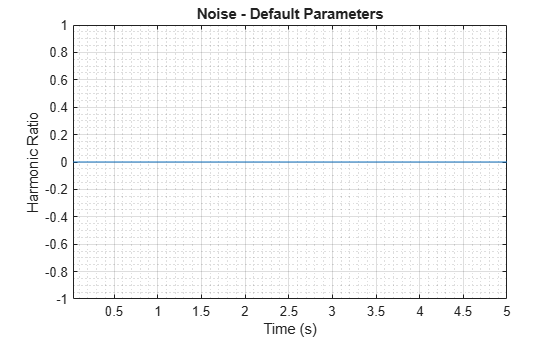## Input Arguments

collapse all

Input signal, specified as a column vector or matrix. If specified as a matrix, `harmonicRatio` treats the columns of the matrix as individual audio channels.

Data Types: `single` | `double`

Sample rate of the input signal in Hz, specified as a positive scalar.

Data Types: `single` | `double`

### Name-Value Pair Arguments

Specify optional comma-separated pairs of `Name,Value` arguments. `Name` is the argument name and `Value` is the corresponding value. `Name` must appear inside quotes. You can specify several name and value pair arguments in any order as `Name1,Value1,...,NameN,ValueN`.

Example: `'Window',hamming(256)`

Window applied in the time domain, specified as the comma-separated pair consisting of '`Window`' and a real vector. The number of elements in the vector must be in the range [1, `size(audioIn,1)`]. The number of elements in the vector must also be greater than `OverlapLength`.

Data Types: `single` | `double`

Number of samples overlapped between adjacent windows, specified as the comma-separated pair consisting of '`OverlapLength`' and an integer in the range [0, `size(Window,1)`).

Data Types: `single` | `double`

## Output Arguments

collapse all

Harmonic ratio, returned as a scalar, vector, or matrix. Each row of `hr` corresponds to the harmonic ratio of a window of `audioIn`. The harmonic ratio is returned with values in the range [0,1]. A value of 0 represents low harmonicity, and a value of 1 represents high harmonicity.

Data Types: `single` | `double`

## Algorithms

The harmonic ratio is calculated as described in . The following algorithm is applied independently to each window of audio data. The normalized autocorrelation of the signal is determined as:

`$\Gamma \left(m\right)=\frac{\sum _{n=1}^{N}s\left(n\right)s\left(n-m\right)}{\sqrt{\sum _{n=1}^{N}s{\left(n\right)}^{2}\sum _{n=0}^{N}s{\left(n-m\right)}^{2}}}\text{\hspace{0.17em}}\text{\hspace{0.17em}}\text{for}\text{\hspace{0.17em}}\left(1\le m\le M\right)$`

where

• s is a single frame of audio data with N elements.

• M is the maximum lag in the calculation. The maximum lag is 40 ms, which corresponds to a minimum fundamental frequency of 25 Hz.

A first estimate of the harmonic ratio is determined as the maximum of the normalized autocorrelation, within a given range:

`$\beta HR=\begin{array}{c}\mathrm{max}\\ {M}_{0}\le m\le M\end{array}\left\{\Gamma \left(m\right)\right\}$`

where M0 is the lower edge of the search range, determined as the first zero crossing of the normalized autocorrelation.

Finally, the harmonic ratio estimate is improved using parabolic interpolation, as described in .

 Kim, Hyoung-Gook, Nicholas Moreau, and Thomas Sikora. MPEG-7 Audio and Beyond: Audio Content Indexing and Retrieval. John Wiley & Sons, 2005.